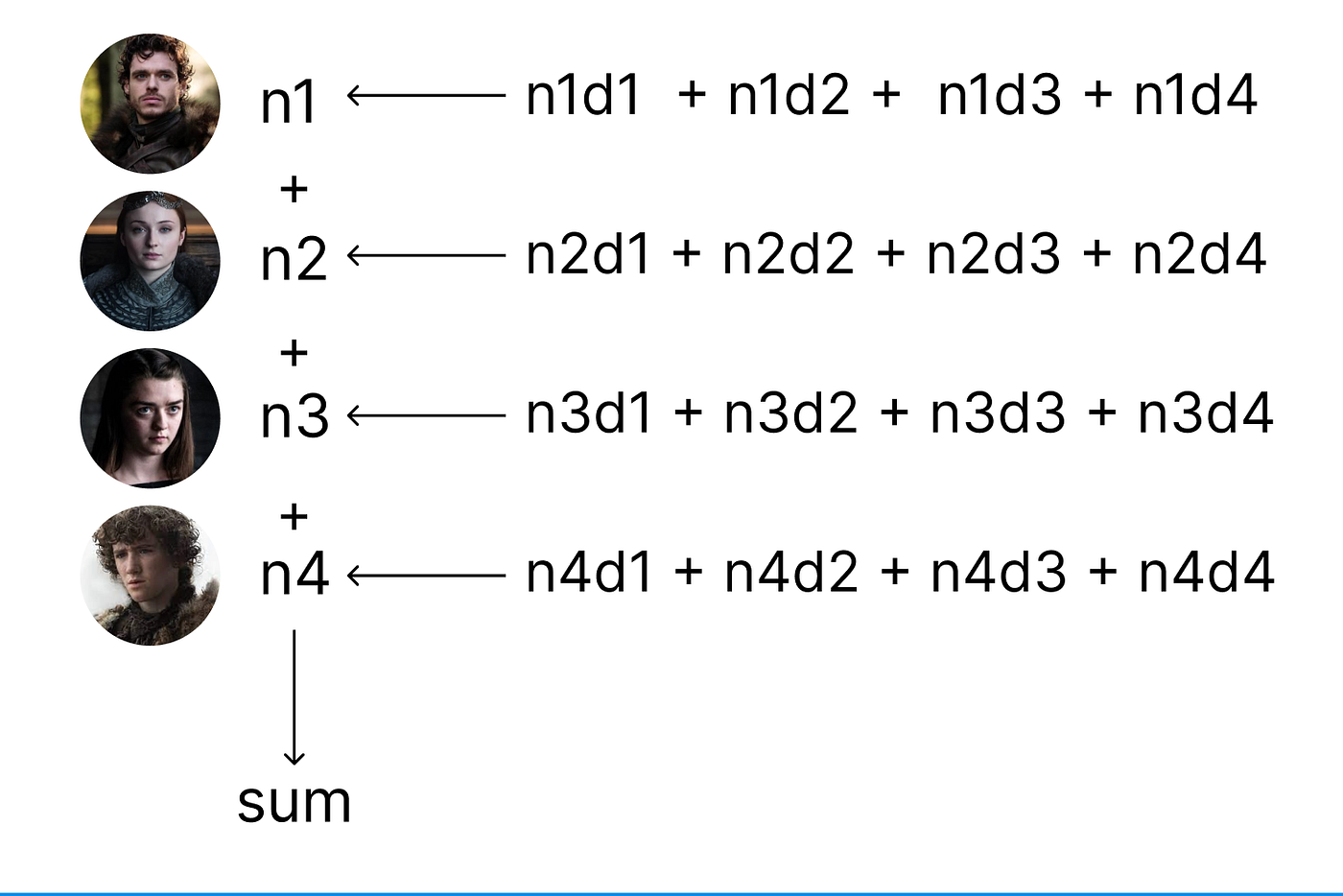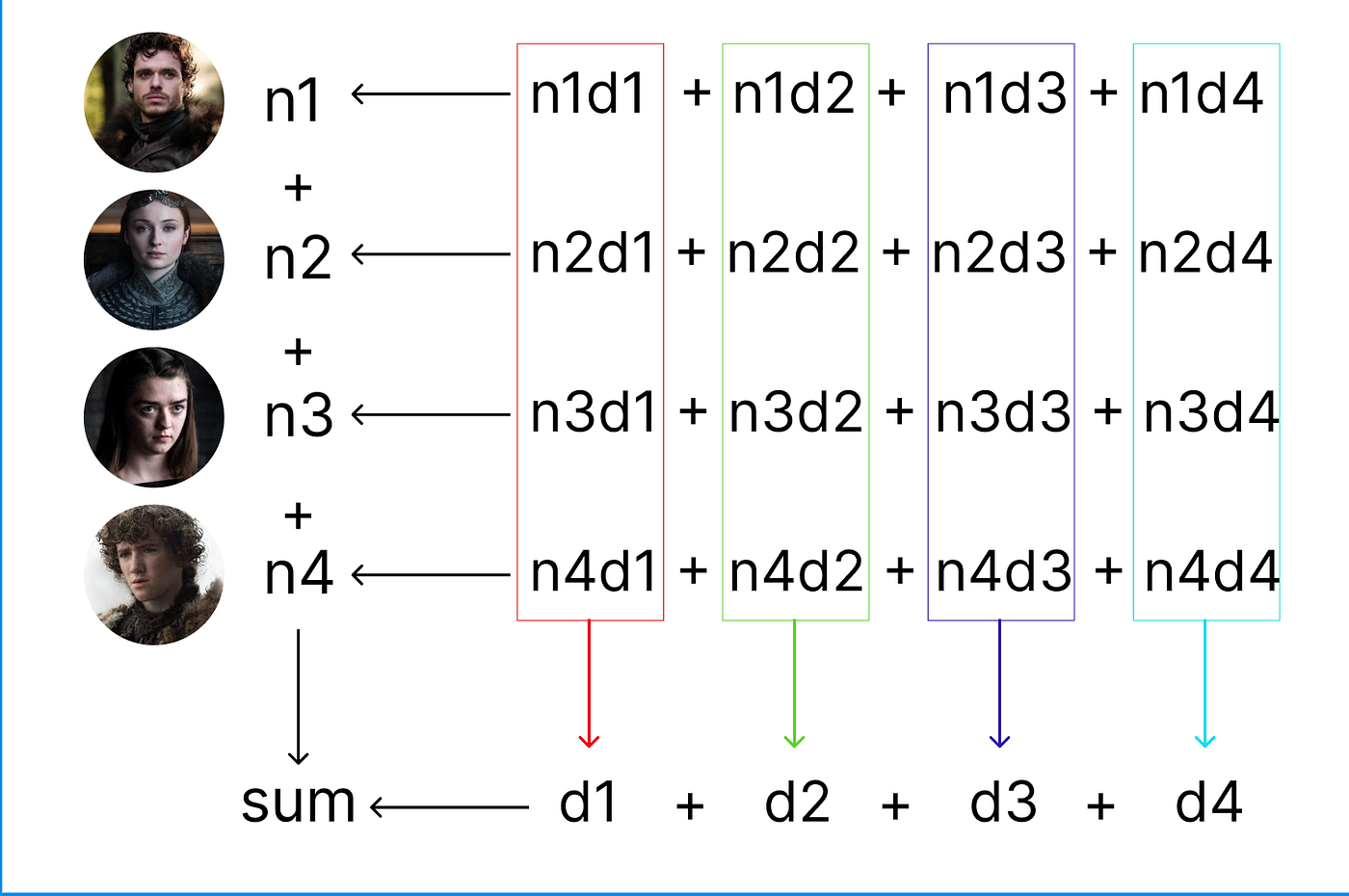# Sarat Angajala's Blog## Understanding multi-party computation, a key piece of technology for next-gen crypto-wallet infrastructure.

Blockchain technology has revolutionized the way we store and transfer digital assets. However, with great power comes great responsibility. One of the biggest challenges facing blockchain users is the need to secure their private keys, which are the digital equivalent of a traditional wallet’s keys. We’ve all heard of cases where losing or exposing these keys resulted in the loss of valuable cryptocurrencies and other digital assets.

To address this challenge, some blockchain wallets use multi-party computation (MPC). MPC allows multiple parties that each hold a fraction of the key to jointly compute the signature without revealing their piece of the key to each other. This means that multiple parties can work together to sign transactions and manage private keys without any one party having access to the complete key. This significantly improves security and reduces the risk of theft or loss.

In this blog, we’ll take a closer look at multi-party computation and explain it using a simple example of adding numbers.

# Problem

Let’s take a simple case where Robb, Sansa, Arya, and Rickon each have a number in their mind. They want to calculate the sum of these four numbers without having to reveal their number to the others.Each person’s number is represented by n1, n2, n3, and n4

# Solution

To arrive at the sum without having to share their individual numbers, each person with come with 4 four random numbers that add up to their initial number and share three of the four numbers with the others so that each person has four segments of the initial numbers.Robb has all the numbers with the d1 suffix, Sansa has all the numbers with the d2 suffix, Arya has all the numbers with the d3 suffix and Rickon has all the numbers with the d4 suffix.

Now each person will add up all the fragments they have and share the sum with the group. These shared sums when added again will give us our desired result.Throughout the process, no single person had access to another person's number. But they were still able to compute the sum keeping their numbers anonymous.

# Mathematical Reasoning

``````required_sum = n1 + n2 + n3 + n4

// expanding each number, we get
required_sum = (n1d1 + n1d2 + n1d3 + n1d4) + (n2d1 + n2d2 + n2d3 + n2d4) + (n3d1 + n3d2 + n3d3 + n3d4) + (n4d1 + n4d2 + n4d3 + n4d4)

// rearranging the numbers into different groups we get
required_sum = (n1d1 + n2d1 + n3d1 + n4d1) + (n1d2 + n2d2 + n3d2 + n4d2) + (n1d3 + n2d3 + n3d3 + n4d3) + (n1d4 + n2d4 + n3d4 + n4d4)

required_sum = d1 + d2 + d3 + d4
``````

# Conclusion

This is the power of MPC. The same concept can be scaled up to use fragments of a private key to sign a message, without one person ever having access to the entire key.

This is the end of today’s article. Any feedback is appreciated.

You can find my socials below if you want to connect.